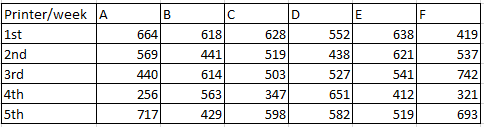### SBI PO 2017 Question 11

Instructions

Study the following table carefully and answer the questions.Question 11

# What is the respective ratio between the number of pages printed by the printer B in 2nd week and the number of pages printed by printer F in 5th week?

Solution

As we can see in the given table that the number of pages printed by printer B in 2nd week is = 441

And number of pages printed by printer F in 5th week is = 693

Hence, the ratio will be = $$\frac{441}{693} = \frac{7}{11}$$